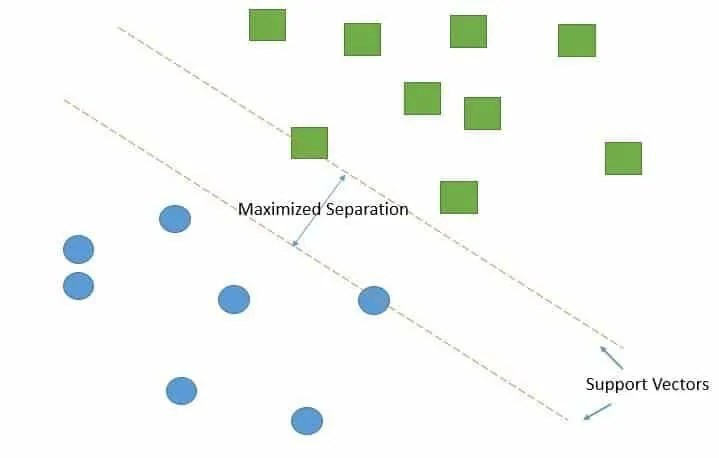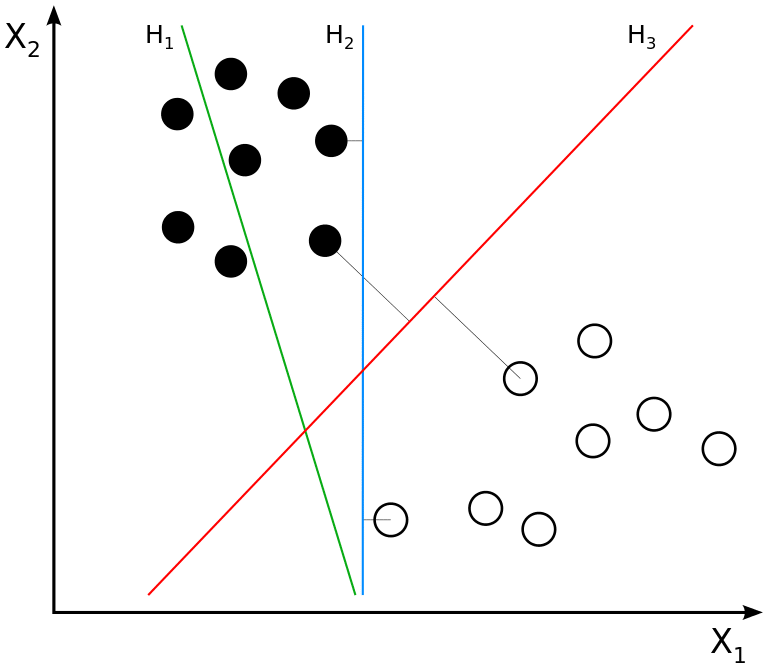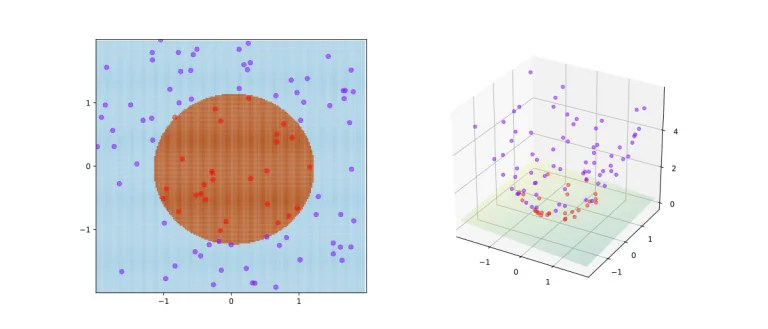# What are Support Vector Machines?Support vector machines are a type of machine learning classifier, arguably one of the most popular kinds of classifiers. Support vector machines are especially useful for numerical prediction, classification, and pattern recognition tasks.

Support vector machines operate by drawing decision boundaries between data points, aiming for the decision boundary that best separates the data points into classes (or is the most generalizable). The goal when using a support vector machine is that the decision boundary between the points is as large as possible so that the distance between any given data point and the boundary line is maximized. That’s a quick explanation of how support vector machines (SVMs) operate, but let’s take some time to delve deeper into how SVMs operate and understand the logic behind their operation.

## The Goal Of Support Vector Machines

Imagine a graph with a number of data points on it, based on features specified by the X and Y axes. The data points on the graph can loosely be divided up into two different clusters, and the cluster that a data point belongs to indicates the class of the data point. Now assume that we want to draw a line down the graph that separates the two classes from each other, with all the data points in one class found on one side of the line and all the data points belonging to another class found on the other side of the line. This separating line is known as a hyperplane.

You can think of a support vector machine as creating “roads” throughout a city, separating the city into districts on either side of the road. All the buildings (data points) that are found on one side of the road belong to one district.The goal of a support vector machine is not only to draw hyperplanes and divide data points, but to draw the hyperplane the separates data points with the largest margin, or with the most space between the dividing line and any given data point. Returning to the “roads” metaphor, if a city planner draws plans for a freeway, they don’t want the freeway to be too close to houses or other buildings. The more margin between the freeway and the buildings on either side, the better. The larger this margin, the more “confident” the classifier can be about its predictions. In the case of binary classification, drawing the correct hyperplane means choosing a hyperplane that is just in the middle of the two different classes. If the decision boundary/hyperplane is farther from one class, it will be closer to another. Therefore, the hyperplane must balance the margin between the two different classes.

## Calculating The Separating Hyperplane From Support Vectors

So how does a support vector machine determine the best separating hyperplane/decision boundary? This is accomplished by calculating possible hyperplanes using a mathematical formula. We won’t cover the formula for calculating hyperplanes in extreme detail, but the line is calculated with the famous slope/line formula:

Y = ax + b

Meanwhile, lines are made out of points, which means any hyperplane can be described as the set of points that run parallel to the proposed hyperplane, as determined by the weights of the model times the set of features modified by a specified offset/bias (“d”).

SVMs draw many hyperplanes. For example, the boundary line is one hyperplane, but the data points that the classifier considers are also on hyperplanes. The values for x are determined based on the features in the dataset. For instance, if you had a dataset with the heights and weights of many people, the “height” and “weight” features would be the features used to calculate the “X”. The margins between the proposed hyperplane and the various “support vectors” (datapoints) found on either side of the dividing hyperplane are calculated with the following formula:

W * X – b

While you can read more about the math behind SVMs, if you are looking for a more intuitive understanding of them just know that the goal is to maximize the distance between the proposed separating hyperplane/boundary line and the other hyperplanes that run parallel to it (and on which the data points are found).## Multiclass Classification

The process described so far applies to binary classification tasks. However, SVM classifiers can also be used for non-binary classification tasks. When doing SVM classification on a dataset with three or more classes, more boundary lines are used. For example, if a classification task has three classes instead of two, two dividing lines will be used to divide up data points into classes and the region that comprises a single class will fall in between two dividing lines instead of one. Instead of just calculating the distance between just two classes and a decision boundary, the classifier must consider now the margins between the decision boundaries and the multiple classes within the dataset.

## Non-Linear Separations

The process described above applies to cases where the data is linearly separable. Note that, in reality, datasets are almost never completely linearly separable, which means that when using an SVM classifier you will often need to use two different techniques: soft margin and kernel tricks. Consider a situation where data points of different classes are mixed together, with some instances belonging to one class in the “cluster” of another class. How could you have the classifier handle these instances?

One tactic that can be used to handle non-linearly separable datasets is the application of a “soft margin” SVM classifier. A soft margin classifier operates by accepting a few misclassified data points. It will try to draw a line that best separates the clusters of data points from each other, as they contain the majority of the instances belonging to their respective classes. The soft margin SVM classifier attempts to create a dividing line that balances the two demands of the classifier: accuracy and margin. It will try to minimize the misclassification while also maximizing the margin.

The SVM’s tolerance for error can be adjusted through manipulation of a hyperparameter called “C”. The C value controls how many support vectors the classifier considers when drawing decision boundaries. The C value is a penalty applied to misclassifications, meaning that the larger the C value the fewer support vectors the classifier takes into account and the narrower the margin.The Kernel Trick operates by applying nonlinear transformations to the features in the dataset. The Kernel Trick takes the existing features in the dataset and creates new features through the application of nonlinear mathematical functions. What results from the application of these nonlinear transformations is a nonlinear decision boundary. Because the SVM classifier is no longer restricted to drawing linear decision boundaries it can start drawing curved decision boundaries that better encapsulate the true distribution of the support vectors and bring misclassifications to a minimum. Two of the most popular SVM nonlinear kernels are Radial Basis Function and Polynomial. The polynomial function creates polynomial combinations of all the existing features, while the Radial Basis Function generates new features by measuring the distance between a central point/points to all the other points.